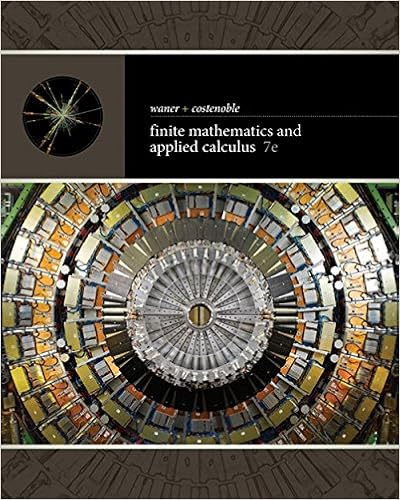# 1536615191-Gr10_Unit 1 test Analytic Geometry (1).pdf -...

• Test Prep
• zahrasupra
• 8

This preview shows page 1 - 3 out of 8 pages.

##### We have textbook solutions for you!
The document you are viewing contains questions related to this textbook.The document you are viewing contains questions related to this textbook.
Chapter 1 / Exercise 121
Finite Mathematics and Applied Calculus
Costenoble/WanerExpert Verified
1Part A: Knowledge/Understanding Identify the choice that best completes the statement or answers the question. Put your answer in the table on page 41. How many solutions are there to the following linear system?
2
2. What are the coordinates of the point where the lines represented in this graph intersect? 3. Which ordered pair is a solution for the linear system and ? a. c. b. d. 4. Translate the following sentence into an equation. “Seven added to eight times a number is three less than twice the number.”a. c. b. d. 5. What is the point of intersection of the linear system and ? a. c. b. d. 6. If Aand Bare points on a grid, the coordinates of the midpoint of segment AB are: a. c. b. d. 14 K/U 11 Comm 13 Think 15 App a. c. b. d. Unit 1 Test Analytic GeometryName ________________________________
##### We have textbook solutions for you!
The document you are viewing contains questions related to this textbook.The document you are viewing contains questions related to this textbook.
Chapter 1 / Exercise 121
Finite Mathematics and Applied Calculus
Costenoble/WanerExpert Verified
2
•••